# More factors, more variance...explained

Risk factor models are at the core of quantitative investing. We’ve been exploring their application within our portfolio series to see if we could create such a model to quantify risk better than using a simplistic volatility measure. That is, given our four portfolios (Satisfactory, Naive, Max Sharpe, and Max Return) can we identify a set of factors that explain each portfolio’s variance relatively well?

In our first investigation, we used the classic Fama-French (F-F) three factor model plus momentum. Results were interesting, but begged the question why we were applying predominantly equity factors to portfolios of diverse assets: bonds, commodities, and real estate in addition to stocks. The simple answer: it was easy to get the data and the factors are already relatively well known.

In our second investigation, we built a risk factor model using economic variables that explained surprisingly little of the four portfolios’ variance. We admit our choice of macro factors wasn’t terribly scientific. But we felt, intuitively, they captured a fair amount of the US economy.1 Unfortunately, the macro factor model did an even worse job explaining portfolio variance than the F-F model.

We hypothesized that some of the problems with the macro factor model—besides data issues around timing and frequency—were that not all of the series we used were leading indicators. In this post, we’ll re-run the model, but with putative leading factors and then see what we can do to improve the model, if at all. Let’s begin!

We start by pulling the data. In the previous post, we used PMI, the unemployment rate, personal consumption expenditures, housing permits, consumer sentiment, and yield spreads (e.g. ten-year less two-year Treasuries, and Baa-rated less Aaa-rated corporates). In this post, we’ll use PMI, initial unemployment claims, the leading index2, housing permits, monetary base, and yield spreads. If these substitutions don’t match your view of true leading indicators, let us know at the email address below. We’re not economists, nor do we pretend to be experts of the dismal science. The variables we use are based on our search into generally accepted leading indicators.

First, here’s the spaghetti graph of the variables transformed to month-over-month sequential changes. We exclude yield spreads since they are not reported as a sequential change, and, if we were to transform them, they would overwhelm the other data.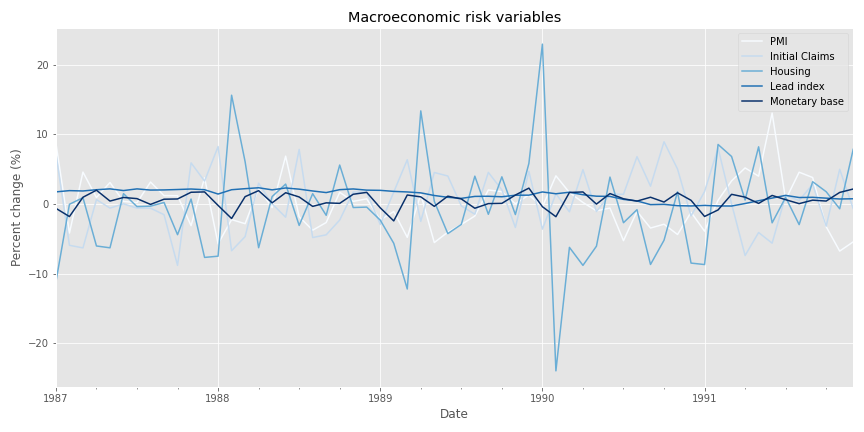Now, we’ll use that data to run our $$R^{2}$$s analysis on the various asset classes.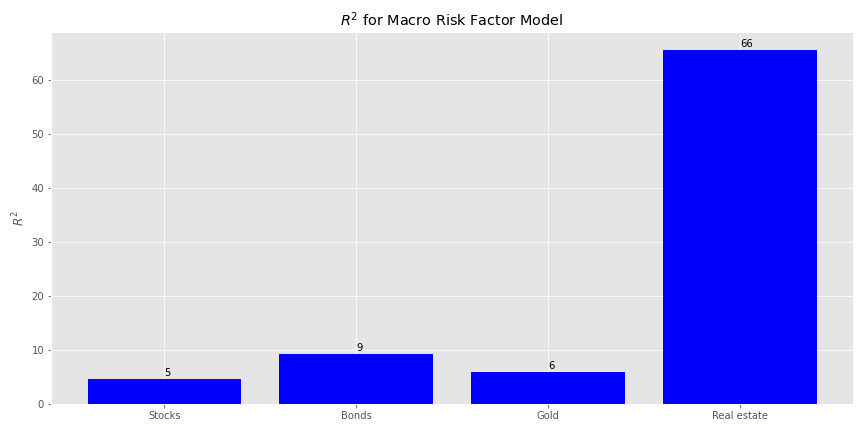Pretty disappointing. The high $$R^{2}$$ for real estate is odd, but we’re not going to dwell on it. We believe working through a full, normalized data series will prove more fruitful. Nonetheless, we’ll show how this macro factor model explains the variance of the four portfolios. We’re skipping over showing how we calculate factor exposures and idiosyncratic risk; you can find that in the code below and the first post. Here’s the explained variance graph.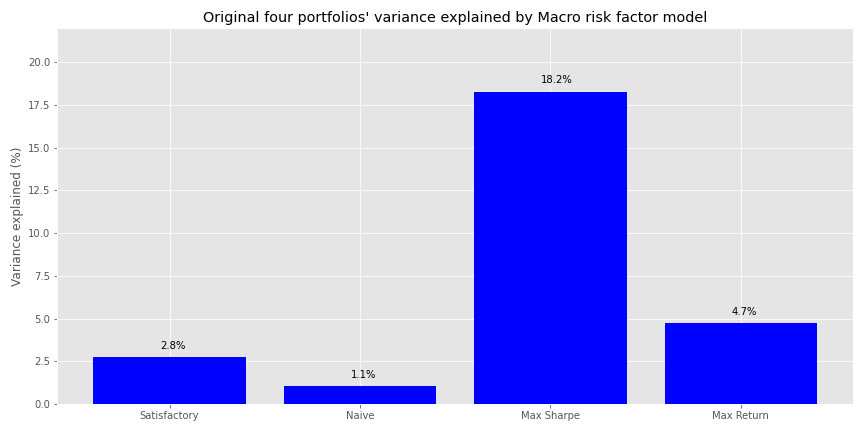This is actually worse than the previous data in terms of explanatory power. Perhaps our intuition wasn’t so bad after all! Let’s move on.

Next we’ll normalize the macro variables and regress them against forward returns. Not knowing which combination of look back and look forward windows is the best, we perform a grid search of rolling three-to-twelve month look back normalizations and one-to-twelve month forward returns, equivalent to 120 different combinations. We sort the results by the highest $$R^{2}$$ for stocks and bonds and show the top five below in a table.

Table 1: Top 5 combinations by $$R^{2}$$ for stocks and bonds
Window Forward Stocks Bonds Gold Real estate
4 8 19.7 8.1 18.0 22.7
5 8 19.4 10.7 14.5 30.2
3 8 18.0 4.9 18.0 18.7
10 5 17.9 12.8 8.1 50.9
7 1 17.0 14.6 16.8 52.8

We’re not sure why three-to-five month look backs on eight month forward returns produce the highest explanatory power. But lets run with the five-month look back and eight month forward (5-8 model). Next we’ll show the factor $$\beta$$s by asset class.Some of the size effects are reasonable in terms of magnitude and direction: monetary base increasing should be positive for stock returns while corporate yield spreads widening should be negative. But others aren’t so much. An increasing monetary base should suggest rising inflation which should be positive for gold. Yet, it appears to have a negative impact for the yellow metal. However, widening corporate spreads, presaging rising risk, should see some correlation with that gilded commodity.

Let’s see how much the 5-8 model explains the variance of the four portfolios.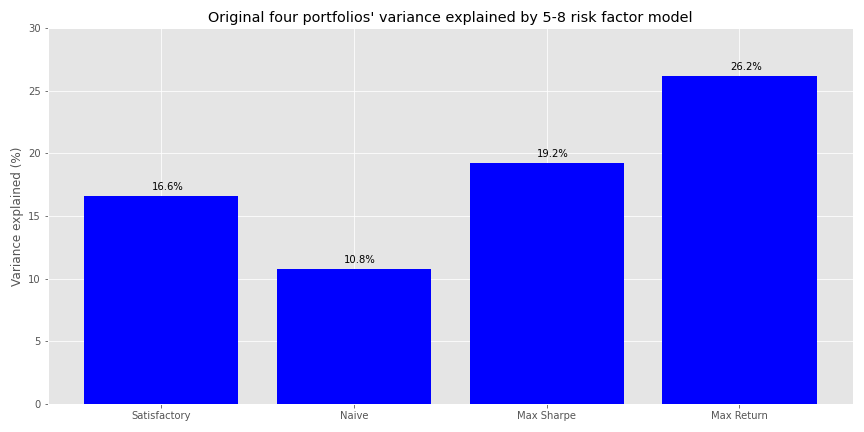Certainly better than the base model and better than the 9-9 model from the previous post. But can we do better?

We’re going to make a broad brush generalization that we won’t try to prove, but we think is generally right. If you think we’re wrong, by all means reach out at the email address below. Here it is. For most investors, most of the time, stocks will be the bulk of the portfolio. They may not be the majority, but they’re likely to be at least a plurality for say 70% of liquid investment portfolios.3 More importantly, unless you’re invested in an alternative asset class or using an arcane investment strategy, stocks will likely produce the bulk of the returns and volatility. Given this gross generalization, perhaps we should include factors that explain stock returns better than the macro variables. Bring back Fama-French!

We add the Fama-French factors to the macro variables and run a grid search as above. Note, we’re excluding the market risk premium as well as risk free rate. Hence, as in the foregoing analysis, we’re analyzing raw returns, rather than risk premia. The grid search table is below.

Table 2: Top 5 combinations by $$R^{2}$$ for stocks and bonds of combined risk factor model
Window Forward Stocks Bonds Gold Real estate
9 5 27.5 14.5 13.1 55.1
10 5 26.9 16.0 14.2 56.4
11 5 26.1 14.5 13.5 55.7
8 5 25.1 12.0 12.4 48.5
12 5 24.6 14.2 15.7 58.1

The look back period clusters around nine to eleven, with returns five-months in the future dominating the top five spots. We’ll go with the ten-month look back and five-month forward (10-5 model).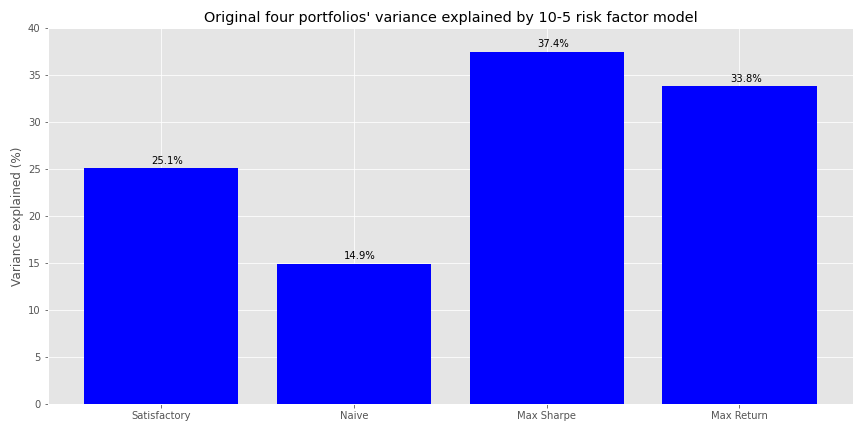Explained variance increased more than five-to-seven percentage points on average with the addition of the F-F factors.

For a final twist, let’s adjust the assets to a risk premium type return (return less the risk free rate) and include the market risk premium. We have to be careful, however. The F-F market risk premium is highly correlated with our stock risk premium.4 So if we included the market risk premium we’d explain almost all of the variance in our stock returns on a coincident basis. On a lagged basis, we’d be incorporating a autoregressive model for stock returns that wouldn’t be present for the other asset classes.

Should we exclude the market risk premium? We think not. Conceptually, the market risk premium is supposed to capture most of the systematic risk not identified by the other factors. This means that even if it is primarily equity market-based, it would likely explain some of the variance of other asset classes, given how much the stock market reflects the overall economy. Hence, it seems reasonable to include the market risk premium in the model, but remove its effect on the stock portion of the portfolio.5Adding the market risk premium and adjusting asset returns to reflect only the amount in excess of the risk-free rate, improved explained portfolio variance for all but the Max Return portfolio. This is encouraging because we worried that removing the risk-free rate might cause some information loss, and hence explanatory power, from raw returns.

We believe the improvement in explained variance for three of the portfolios is due mainly to an increase in the risk model’s power to explain bond returns. While we don’t show it, the $$R^{2}$$ for bonds increased about seven percentage points by moving from the raw return to the market risk premium model. Recall that only the Max Return portfolio has a small exposure to bonds, while the others are above 20%. So maybe the market risk premium for equities does confer some information for bonds. Many companies are in both buckets after all.

How does this all compare to the original model using only F-F? On first blush, not so well. While the Max Sharpe portfolio saw a huge jump in explanatory power, the remaining portfolios saw modest changes or actual declines. Here’s the graph of that original output.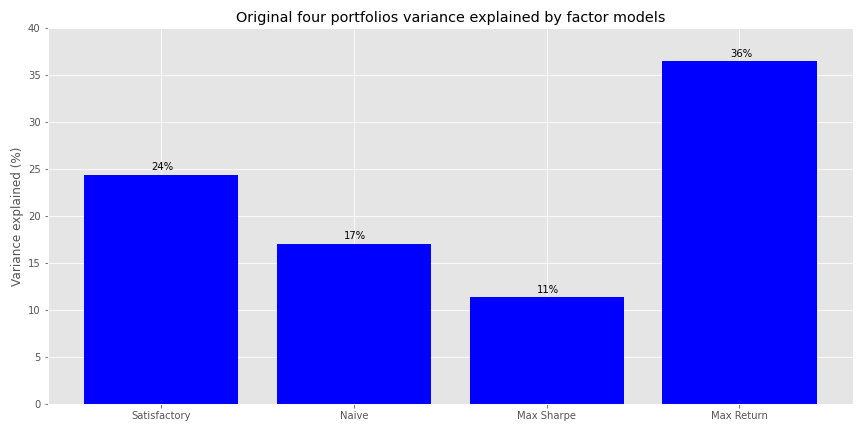Of course, that original model was on a coincident time scale, so it does little to help us forecast impending risk. Clearly, the addition of macro variables helped the Max Sharpe portfolio because it had a very high weighting to real estate, whose variance in returns was explained best by the macro and macro plus F-F models. Nonetheless, we don’t think we should be discouraged by the results. Quite the opposite. That we were able to maintain the magnitude of variance explained on forward returns, which are notoriously difficult to forecast anyway, may commend the use of macro variables.

This isn’t time to take a victory lap, however. Many open questions about the model remain. First, while not a (insert risk factor commercial provider here) 40 factor model, with 11 factors our model isn’t exactly parsimonious. Second, we arbitrarily chose a look back/look forward combination we liked. There wasn’t anything rigorous to it and there’s no evidence, without out-of-sample validation, that the model would generalize well on unseen data. Third, even with all this data wrangling, we still haven’t found a model that explains even half of the variance of the portfolios. True, it’s better than zero, but that begs the question as to which matters more: behavior or opportunity cost. That is, while the simplistic risk measure volatility doesn’t tell us much about the future, it does tell us that returns will vary greatly. The risk factor models tell us that some of the future variance may be explained by some factors we’ve observed today, but the majority of the variance is still unknown.

Are you more comfortable knowing that you won’t know or knowing that you’ll know some, but not a lot and it will cost you time (and/or money) to get there? With that open question we’ll end this post. And now for the best part… the code!

# Built with R 4.0.3 and Python 3.8.3

#[R code]
## Load packages
suppressPackageStartupMessages({
library(tidyverse)
library(tidyquant)
library(reticulate)
})

# Allow variables in one python chunk to be used by other chunks.
knitr::knit_engines$set(python = reticulate::eng_python) # [Python code] # Load libraries import warnings warnings.filterwarnings('ignore') import numpy as np import pandas as pd import statsmodels.api as sm import matplotlib import matplotlib.pyplot as plt import os os.environ['QT_QPA_PLATFORM_PLUGIN_PATH'] = 'C:/Users/nbwal/Anaconda3/Library/plugins/platforms' plt.style.use('ggplot') DIR = "C:/Users/user/image_folder" # Whatever directory you want to save the graphs in. This is the static folder in blogdown so we can grab png files instead running all of the code every time we want to update the Rmarkdown file. ## Create save figure function def save_fig_blog(fig_id, tight_layout=True, fig_extension="png", resolution=300): path = os.path.join(DIR, fig_id + "." + fig_extension) print("Saving figure", fig_id) if tight_layout: plt.tight_layout() plt.savefig(path, format=fig_extension, dip=resolution) ## Random portfolios for later # See other posts for Port_sim.calc_sim_lv() function np.random.seed(42) port1, wts1, sharpe1 = Port_sim.calc_sim_lv(df = df.iloc[1:60, 0:4], sims = 10000, cols=4) ## Download and wrangle macro variables import quandl quandl.ApiConfig.api_key = 'your_key' start_date = '1970-01-01' end_date = '2019-12-31' pmi = quandl.get("ISM/MAN_PMI", start_date=start_date, end_date=end_date) pmi = pmi.resample('M').last() # PMI is released on first of month. But most of the other data is on the last of the month start_date = '1970-01-01' end_date = '2019-12-31' indicators = ['ICSA', 'PERMIT','USSLIND', 'BOGMBASE', 'T10Y2Y', 'DAAA', 'DBAA'] fred_macros = {} for indicator in indicators: fred_macros[indicator] = dr.DataReader(indicator, 'fred', start_date, end_date) fred_macros[indicator] = fred_macros[indicator].resample('M').last() from functools import reduce risk_factors = pmi for indicator in indicators: risk_factors = pd.merge(risk_factors, fred_macros[indicator], how = 'left', left_index=True, right_index=True) risk_factors['CORP'] = risk_factors['DBAA'] - risk_factors['DAAA'] risk_factors = risk_factors.drop(['DAAA', 'DBAA'], axis=1) # Transform to sequential change macro_risk_chg = risk_factors.copy() macro_risk_chg.loc[:, ['PMI','ICSA', 'PERMIT', 'BOGMBASE']] = macro_risk_chg.loc[:, ['PMI','ICSA', 'PERMIT', 'BOGMBASE']].pct_change() macro_risk_chg.loc[:, ['USSLIND','T10Y2Y', 'CORP']] = macro_risk_chg.loc[:, ['USSLIND','T10Y2Y', 'CORP']].apply(lambda x: x*.01) ## Remove infinites for OLS macro_risk_chg = macro_risk_chg.replace([np.inf, -np.inf], 0.0) ## Spaghetti graph of macro variables ax = (macro_risk_chg.loc['1987':'1991', macro_risk_chg.columns[:-2].to_list()]*100).plot(figsize=(12,6), cmap = 'Blues') # macro_risk_chg.loc['1987':'1991', macro_risk_chg.columns[-2:].to_list()].plot(ax = ax) # Include only if want yield spreads too plt.legend(['PMI', 'Initial Claims', 'Housing', 'Leading indicators', 'Monetary base']) plt.ylabel('Percent change (%)') plt.title('Macroeconomic risk variables') plt.show() ## Create function to calculate R-squareds, with or without risk premiums, graph them, and do a whole bunch of other stuff that makes analysis and blog writing easier import statsmodels.api as sm ## Load data risk_factors = pd.read_pickle('risk_factors_2.pkl') df = pd.read_pickle('port_const.pkl') df.iloc[0,3] = 0.006 dep_df_all = df.iloc[:,:-1] ## Create RSQ function # Allows one to remove market risk premium from stock regression def rsq_func_prem(ind_df, dep_df, look_forward = None, risk_premium = False, period = 60, start_date=0, \ plot=True, asset_names = True, print_rsq = True, chart_title = None,\ y_lim = None, save_fig = False, fig_name = None): """ Assumes ind_df starts from the same date as dep_df. Dep_df has only as many columns as interested for modeling. """ xs = ind_df[0:start_date+period] assets = dep_df.columns.to_list() rsq = [] if look_forward: start = start_date + look_forward end = start_date + look_forward + period else: start = start_date end = start_date + period if risk_premium: mask = [x for x in factors.columns.to_list() if x != 'mkt-rfr'] for asset in assets: if asset == 'stock': X = sm.add_constant(xs.loc[:,mask]) else: X = sm.add_constant(xs) y = dep_df[asset][start:end].values mod = sm.OLS(y, X).fit().rsquared*100 rsq.append(mod) if print_rsq: print(f'R-squared for {asset} is {mod:0.03f}') else: X = sm.add_constant(xs) for asset in assets: y = dep_df[asset][start:end].values mod = sm.OLS(y, X).fit().rsquared*100 rsq.append(mod) if print_rsq: print(f'R-squared for {asset} is {mod:0.03f}') if plot: if asset_names: x_labels = ['Stocks', 'Bonds', 'Gold', 'Real estate'] else: x_labels = asset_names plt.figure(figsize=(12,6)) plt.bar(x_labels, rsq, color='blue') for i in range(4): plt.annotate(str(round(rsq[i]),), xy = (x_labels[i], rsq[i]+0.5)) plt.ylabel("$R^{2}$") if chart_title: plt.title(chart_title) else: plt.title("$R^{2}$for Macro Risk Factor Model") plt.ylim(y_lim) if save_fig: save_fig_blog(fig_name) else: plt.tight_layout() plt.show() return rsq # Run r-squared function import statsmodels.api as sm cols = macro_risk_chg.columns[:-2].to_list() ind_df_1 = macro_risk_chg.loc['1987':'1991',cols] dep_df_1 = df.iloc[:60,:4] _ = rsq_func_prem(ind_df_1, dep_df_1, y_lim = None, save_fig = True, fig_name = 'macro_risk_r2_22') ## Create factor beta calculation function ## while allowing for market risk premium exposure def factor_beta_risk_premium_calc(ind_df, dep_df, risk_premium = False): """ Assumes only necessary columns in both ind_df and dep_df data frames. Set risk_premium to True if you include the market risk premium in ind_df and don't want to regress stock returns against i. """ xs = ind_df factor_names = [x.lower() for x in ind_df.columns.to_list()] assets = dep_df.columns.to_list() betas = pd.DataFrame(index=dep_df.columns) pvalues = pd.DataFrame(index=dep_df.columns) error = pd.DataFrame(index=dep_df.index) if risk_premium: mask = [x for x in ind_df.columns.to_list() if x != 'mkt-rfr'] # remove market risk premium from independent variables zero_val = np.where(ind_df.columns == 'mkt-rfr') # identify index of market risk premium for asset in assets: if asset == 'stock': X = sm.add_constant(xs.loc[:,mask]) y = dep_df[asset].values result = sm.OLS(y, X).fit() # pad results for missing market risk premium results = np.array([x for x in result.params[1:zero_val+1]] + [0.0] + [x for x in result.params[zero_val+1:]]) for j in range(len(results)): # results and factor names have same length betas.loc[asset, factor_names[j]] = results[j] pvalues.loc[asset, factor_names[j]] = results[j] else: X = sm.add_constant(xs) y = dep_df[asset].values result = sm.OLS(y, X).fit() for j in range(1, len(result.params)): # result.params equals length of factor_names + 1 due to intercept so start at 1 betas.loc[asset, factor_names[j-1]] = result.params[j] pvalues.loc[asset, factor_names[j-1]] = result.pvalues[j] # Careful of error indentation: lopping through assets error.loc[:,asset] = (y - X.dot(result.params)) else: X = sm.add_constant(xs) for asset in assets: y = dep_df[asset].values result = sm.OLS(y, X).fit() for j in range(1, len(result.params)): betas.loc[asset, factor_names[j-1]] = result.params[j] pvalues.loc[asset, factor_names[j-1]] = result.pvalues[j] error.loc[:,asset] = (y - X.dot(result.params)) return betas, pvalues, error ## Create function to plot betas def betas_plot(beta_df, colors, legend_names, save_fig = False, fig_name=None): beta_df.plot(kind='bar', width = 0.75, color= colors, figsize=(12,6)) plt.legend(legend_names) plt.xticks([0,1,2,3], ['Stock', 'Bond', 'Gold', 'Real estate'], rotation=0) plt.ylabel(r'Factor$\beta$s') plt.title(r'Factor$\beta$s by asset class') if save_fig: save_fig_blog(fig_name) plt.show() ## Create function to calculate variance due to risk factors def factor_port_var(betas, factors, weights, error): B = np.array(betas) F = np.array(factors.cov()) S = np.diag(np.array(error.var())) factor_var = weights.dot(B.dot(F).dot(B.T)).dot(weights.T) specific_var = weights.dot(S).dot(weights.T) return factor_var, specific_var ## Create weight variables to calculate portfolio explained variance satis_wt = np.array([0.32, 0.4, 0.2, 0.08]) equal_wt = np.repeat(0.25,4) max_sharp_wt = wts1[np.argmax(sharpe1)] max_ret_wt = wts1[pd.DataFrame(np.c_[port1,sharpe1], columns = ['ret', 'risk', 'sharpe']).sort_values(['ret', 'sharpe'], ascending=False).index] ## Calculate portfolio variance wt_list = [satis_wt, equal_wt, max_sharp_wt, max_ret_wt] port_exp=[] betas1, pvalues1, error1 = factor_beta_risk_premium_calc(ind_df_1, dep_df_1) for wt in wt_list: out = factor_port_var(betas1, ind_df_1, wt, error1) port_exp.append(out/(out + out)) port_exp = np.array(port_exp) ## Create function to plot portfolio explained variance def port_var_plot(port_exp, port_names=None, y_lim=None, save_fig=False, fig_name=None): if not port_names: port_names = ['Satisfactory', 'Naive', 'Max Sharpe', 'Max Return'] else: port_names = port_names plt.figure(figsize=(12,6)) plt.bar(port_names, port_exp*100, color='blue') for i in range(4): plt.annotate(str(round(port_exp[i]*100,1)) + '%', xy = (i-0.05, port_exp[i]*100+0.5)) plt.title('Original four portfolios variance explained by Macro risk factor model') plt.ylabel('Variance explained (%)') plt.ylim(y_lim) if save_fig: save_fig_blog(fig_name) plt.show() # Graph portfolio variance based on macro model port_var_plot(port_exp, y_lim = [0,22]) ## Run grid search based on look back normalization and look forward returns dep_df_all = df.iloc[:,:-1] # Grid search for best params scale_for = pd.DataFrame(np.c_[np.array([np.repeat(x,12) for x in range(3,13)]).flatten(),\ np.array([np.arange(1,13)]*10).flatten(),\ np.array([np.zeros(120)]*4).T],\ columns = ['Window', 'Forward', 'Stocks', 'Bonds', 'Gold', 'Real estate']) count = 0 for i in range(3, 13): risk_scale = risk_factors.apply(lambda x: (x - x.rolling(i).mean())/x.rolling(i).std(ddof=1))['1987':] risk_scale.replace([np.inf, -np.inf], 0.0, inplace=True) for j in range(1,13): out = rsq_func_prem(risk_scale, dep_df_all, look_forward = j,\ plot=False,print_rsq=False) scale_for.iloc[count,2:] = np.array(out) count+=1 ## Sort data scaled_sort = scale_for.sort_values(['Stocks','Bonds'], ascending=False).round(1).reset_index() # [R] ## Print table py$scaled_sort %>% as.data.frame(check.names=FALSE) %>%
select(-index) %>%
slice(1:5) %>%
knitr::kable('html', caption = "Top 5 combinations by $R^{2}$ for stocks and bonds")

## Build model based on grid search model using 5-8 look back/look forward
scale_5_8 = risk_factors.apply(lambda x: (x - x.rolling(5).mean())/x.rolling(5).std(ddof=1))['1987':]
scale_5_8.replace([np.inf, -np.inf], 0.0, inplace=True)
_ = rsq_func_prem(scale_5_8, dep_df_all, look_forward = 8, y_lim = [0,35],save_fig=False)

betas_scale, _, error_scale = factor_beta_risk_premium_calc(scale_5_8['1987':'1991'],df.iloc[8:68,:-1])

beta_colors = ['darkblue', 'darkgrey', 'blue', 'grey','lightblue', 'lightgrey', 'black']
beta_names = ['PMI', 'Initial claims', 'Housing','Leading indicators', 'Monetary base', 'Treasury', 'Corp']
betas_plot(betas_scale, beta_colors, beta_names, save_fig=True, fig_name = 'factor_betas_5_8_22')

## Graph portfolio explained variance based on 5-8 grid search model
satis_wt = np.array([0.32, 0.4, 0.2, 0.08])
equal_wt = np.repeat(0.25,4)
max_sharp_wt = wts1[np.argmax(sharpe1)]
max_ret_wt = wts1[pd.DataFrame(np.c_[port1,sharpe1], columns = ['ret', 'risk', 'sharpe']).sort_values(['ret', 'sharpe'], ascending=False).index]

wt_list = [satis_wt, equal_wt, max_sharp_wt, max_ret_wt]
port_exp=[]

for wt in wt_list:
out = factor_port_var(betas_scale, scale_5_8['1987':'1991'], wt, error_scale)
port_exp.append(out/(out + out))

port_exp = np.array(port_exp)

port_var_plot(port_exp, y_lim=[0,30], save_fig=True, fig_name='four_port_var_exp_5_8_22')

## Load Fama-French Factors
try:
ff_mo = pd.read_pickle('ff_mo.pkl')
print('Data loaded')
except FileNotFoundError:
print("File not found")
print('Loading data yo...')
ff_url = "http://mba.tuck.dartmouth.edu/pages/faculty/ken.french/ftp/F-F_Research_Data_Factors_CSV.zip"
col_names = ['date', 'mkt-rfr', 'smb', 'hml', 'rfr']
ff = pd.read_csv(ff_url, skiprows=3, header=0, names = col_names)
ff = ff.iloc[:1132,:]
from pandas.tseries.offsets import MonthEnd
ff['date'] = pd.to_datetime([str(x[:4]) + "/" + str.rstrip(x[4:]) for x in ff['date']], format = "%Y-%m") + MonthEnd(1)
ff.iloc[:,1:] = ff.iloc[:,1:].apply(pd.to_numeric)
momo_url = "http://mba.tuck.dartmouth.edu/pages/faculty/ken.french/ftp/F-F_Momentum_Factor_CSV.zip"
momo = pd.read_csv(momo_url, skiprows=13, header=0, names=['date', 'mom'])
momo = momo.iloc[:1125,:]
momo['date'] = pd.to_datetime([str(x[:4]) + "/" + str(x[4:]) for x in momo['date']], format = "%Y-%m") + MonthEnd(1)
momo['mom'] = pd.to_numeric(momo['mom'])
ff_mo = pd.merge(ff, momo, how = 'left', on='date')
col_ord = [x for x in ff_mo.columns.to_list() if x not in ['rfr']] + ['rfr']
ff_mo = ff_mo.loc[:,col_ord]

ff_mo.set_index('date', inplace=True)
ff_mo = ff_mo*.01 # Convert percentages to decimal. IMPORTANT FOR RISK PREMIUM!!

# Create total factors model
total_factors = pd.merge(risk_factors, ff_mo, how='left', left_index=True, right_index=True)

## Grid search for best params

# Prepare data
dep_df_all = df.iloc[:,:-1]

# Risk factor data
keep = [x for x in total_factors.columns.to_list() if x not in ['mkt-rfr', 'rfr']]
risk_factors_1 = total_factors.loc[:, keep]

scale_for = pd.DataFrame(np.c_[np.array([np.repeat(x,12) for x in range(3,13)]).flatten(),\
np.array([np.arange(1,13)]*10).flatten(),\
np.array([np.zeros(120)]*4).T],\
columns = ['Window', 'Forward', 'Stocks', 'Bonds', 'Gold', 'Real estate'])
count = 0
for i in range(3, 13):
risk_scale = risk_factors_1.apply(lambda x: (x - x.rolling(i).mean())/x.rolling(i).std(ddof=1))['1987':]
risk_scale.replace([np.inf, -np.inf], 0.0, inplace=True)
for j in range(1,13):
out = rsq_func_prem(risk_scale, dep_df_all, look_forward = j, plot=False, print_rsq=False)
scale_for.iloc[count,2:] = np.array(out)
count+=1

scaled_sort = scale_for.sort_values(['Stocks', 'Bonds'], ascending=False).round(1).reset_index()

[R]
## Print table from Python data frame
py$scaled_sort %>% as.data.frame(check.names=FALSE) %>% select(-index) %>% slice(1:5) %>% knitr::kable('html', caption = "Top 5 combinations by$R^{2}\$ for stocks and bonds of combined risk factor model")

## Build grid search model and graph portfolio variance
scale_10_5 = risk_factors_1.apply(lambda x: (x - x.rolling(10).mean())/x.rolling(10).std(ddof=1))['1987':]
scale_10_5.replace([np.inf, -np.inf], 0.0, inplace=True)

betas_10_5, _, error_10_5 = factor_beta_risk_premium_calc(scale_10_5['1987':'1991'],df.iloc[5:65,:-1])

satis_wt = np.array([0.32, 0.4, 0.2, 0.08])
equal_wt = np.repeat(0.25,4)
max_sharp_wt = wts1[np.argmax(sharpe1)]
max_ret_wt = wts1[pd.DataFrame(np.c_[port1,sharpe1], columns = ['ret', 'risk', 'sharpe']).sort_values(['ret', 'sharpe'], ascending=False).index]

wt_list = [satis_wt, equal_wt, max_sharp_wt, max_ret_wt]
port_exp=[]

for wt in wt_list:
out = factor_port_var(betas_10_5, scale_10_5['1987':'1991'], wt, error_10_5)
port_exp.append(out/(out + out))

port_exp = np.array(port_exp)

port_var_plot(port_exp, y_lim=[0,40], save_fig=True, fig_name = 'four_port_var_exp_10_5_22')

## Run gird search for risk premium and market-risk premium factor model
### Grid search for best params

## Prepare data

# Risk premium data
dep_df_all = df.iloc[:,:-1].apply(lambda x: x - total_factors.loc['1987':'2019','rfr'].values)

# Risk factor data
risk_factors_3 = total_factors.loc[:, total_factors.columns.to_list()[:-1]]

## Create data frame
scale_for = pd.DataFrame(np.c_[np.array([np.repeat(x,12) for x in range(3,13)]).flatten(),\
np.array([np.arange(1,13)]*10).flatten(),\
np.array([np.zeros(120)]*4).T],\
columns = ['Window', 'Forward', 'Stocks', 'Bonds', 'Gold', 'Real estate'])
## Iterate
count = 0
for i in range(3, 13):
risk_scale = risk_factors_3.apply(lambda x: (x - x.rolling(i).mean())/x.rolling(i).std(ddof=1))['1987':]
risk_scale.replace([np.inf, -np.inf], 0.0, inplace=True)
for j in range(1,13):
out = rsq_func_prem(risk_scale, dep_df_all, risk_premium = True, look_forward = j, plot=False, print_rsq=False)
scale_for.iloc[count,2:] = np.array(out)
count+=1

scale_10_5 = risk_factors_3.apply(lambda x: (x - x.rolling(10).mean())/x.rolling(10).std(ddof=1))['1987':]
scale_10_5.replace([np.inf, -np.inf], 0.0, inplace=True)
_ = rsq_func_prem(scale_10_5, dep_df_all, look_forward = 5, risk_premium = True, y_lim = [0,60],save_fig=False)

betas_10_5a, _, error_10_5a = factor_beta_risk_premium_calc(scale_10_5['1987':'1991'],dep_df_all.iloc[5:65,:], risk_premium=True)

satis_wt = np.array([0.32, 0.4, 0.2, 0.08])
equal_wt = np.repeat(0.25,4)
max_sharp_wt = wts1[np.argmax(sharpe1)]
max_ret_wt = wts1[pd.DataFrame(np.c_[port1,sharpe1], columns = ['ret', 'risk', 'sharpe']).sort_values(['ret', 'sharpe'], ascending=False).index]

wt_list = [satis_wt, equal_wt, max_sharp_wt, max_ret_wt]
port_exp=[]

for wt in wt_list:
out = factor_port_var(betas_10_5a, scale_10_5['1987':'1991'], wt, error_10_5a)
port_exp.append(out/(out + out))

port_exp = np.array(port_exp)

port_var_plot(port_exp, y_lim=[0,42], save_fig=True, fig_name='four_port_var_10_5_rp_22')

1. We apologize to our non-US readers. The US-bias is data-driven not philosophical. If any kind soul knows of easy to procure international data of sufficient length and frequency (i.e., more than 30 years and at least monthly), please let us know at content at optionstocksmachines.com. We’ll be happy to incorporate it into a post or three!↩︎

2. From the FRED website: “The leading index for each state predicts the six-month growth rate of the state’s coincident index. In addition to the coincident index, the models include other variables that lead the economy: state-level housing permits (1 to 4 units), state initial unemployment insurance claims, delivery times from the Institute for Supply Management (ISM) manufacturing survey, and the interest rate spread between the 10-year Treasury bond and the 3-month Treasury bill.” The perspicuous reader will note that there is some double counting since we also include housing permits in the model. However, when we used only the leading index, we didn’t find much to commend the model. Hopefully, the impact of permits in the leading index is modest.↩︎

3. Around 70% of the world’s population lives in developed countries and this is the main investing population. Of the more developed countries over 50% of the population is in the 25-64 years old range, which represents say 90% of investors. And if the most often recommended portfolio is some combination of 80/20 to 60/40 stocks/bonds, then 50-70% of portfolios will be overweight stocks.↩︎

4. We’re using the Wilshire 5000 total return index.↩︎

5. This is, of course, easy enough to do if we were just writing procedural code; writing functional code, with proper evaluators to ensure successful matrix multiplication is where it becomes a bit more gnarly!↩︎